Courses

# Water Demand Notes | EduRev

## Civil Engineering (CE) : Water Demand Notes | EduRev

The document Water Demand Notes | EduRev is a part of the Civil Engineering (CE) Course Environmental Engineering.
All you need of Civil Engineering (CE) at this link: Civil Engineering (CE)

Fire Demand

Rate of fire demand is sometimes treated as a function of population and is worked out on the basis of empirical formulas:

1. As per GO Fire Demand
= 100(P)1/2
2. Kuichling’s Formula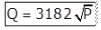Where Q = Amount of water required in liters/minute.
P = Population in thousand.
3. Freeman Formula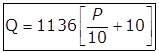4. National Board of Fire Under Writers Formula
(i) For a central congested high valued city
(a) Where population < 200000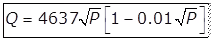(b) where population > 200000
Q = 54600 lit/minute for first fire
and Q = 9100 to 36,400 lit/minute for a second fire.
(ii) For a residential city.
(a) Small or low building,
Q = 2,200 lit/minutes.
(b) Larger or higher buildings,
Q = 4500 lit/minute.
(c) High value residences, apartments, tenements
Q = 7650 to 13,500 lit/minute.
(d) Three storeyed buildings in density built-up sections,
Q = 27000 lit/minute.
5. Buston’s Formula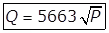The probability of occurrence of a fire, which, in turn, depends upon the type of the city served, has been taken into consideration in developing a above formula on the basis of actual water consumption in fire fighting for Jabalpur city of India. The formula is given as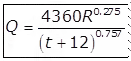Where,
R = Recurrence interval of fire i.e., period of occurrence of fire in years, which will be different for residential, commercial and industrial cities.

Per Capita Demand (q)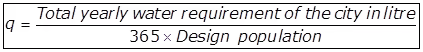Assessment of Normal Variation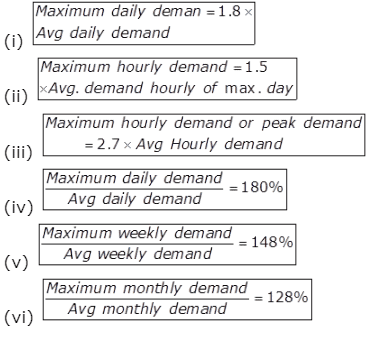Population forecasting Methods
1. Arithmetic increase method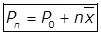Where,
Prospective or forecasted population after n decades from the present (i.e., last known census)
Population at present (i.e., last known census)
n = Number of decades between now & future.
Average (arithmetic mean) of population increases in the known decades.
2. Geometric Increase Method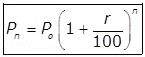where,
Po = Initial population.
Pn = Future population after ‘n’ decades.
r = Assumed growth rate (%).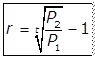where,
P2 = Final known population
P1 = Initial known population
t = Number of decades (period) between P1 and P2.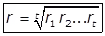3. Incremental Increases Method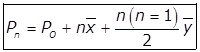Where,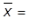Average increase of population of known decades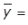Average of incremental increases of the known decades.
4. Decreasing rate of growth method
Since the rate of increase in the population goes on reducing, as the cities reach towards saturation, a method which makes use of the decrease in the percentage increase, in many times used, and gives quite rational results. In this method, the average decrease in the percentage increase is worked out, and is then subtracted from the latest percentage increase for each successive decade. This method is, however, applicable only in cases, where the rate of growth shows a downward trend.
5. Logistic Curve Method
(a)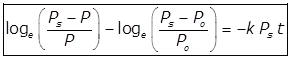Where,
Po = Population of the start point.
Ps = Saturation population
P = Population at any time t from the origin.
k = Constant.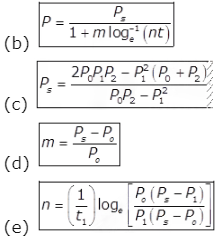Offer running on EduRev: Apply code STAYHOME200 to get INR 200 off on our premium plan EduRev Infinity!

## Environmental Engineering

14 videos|73 docs|70 tests

,

,

,

,

,

,

,

,

,

,

,

,

,

,

,

,

,

,

,

,

,

;